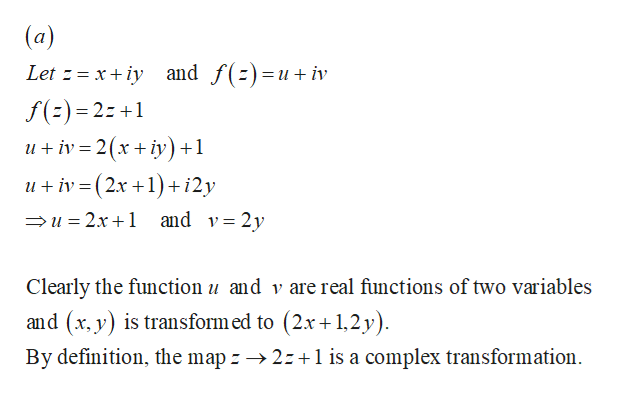are the following mappings transformations of the complex plane:(a). z --> 2z+1(b). x + yi --> x^2+y^2i

Question

are the following mappings transformations of the complex plane:

(a). z --> 2z+1

(b). x + yi --> x^2+y^2i

Step 1

A complex transformation is a mapping on the complex plane ...help_outlineImage Transcriptionclose(a) and f()=u+ iv Let xiy f() 2: + ui 2(xy) +1 uiv(2x 1+ i2y u 2x1 and v= 2y Clearly the function u and v are real functions of two variables is transform ed to (2x1,2y) By definition, the map 2z1 is a complex transformation and (x, fullscreen

Want to see the full answer?

See Solution

Want to see this answer and more?

Our solutions are written by experts, many with advanced degrees, and available 24/7

See Solution
Tagged in

3D Geometry